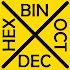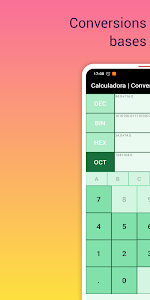# Binary Calculator Hexadecimal to decimal converter v5.0

Downloads: 1283

Free

### Binary Calculator Hexadecimal to decimal converter / Screenshots### Binary Calculator Hexadecimal to decimal converter / Description

Binary Calculator hexadecimal octal and decimal converter & translator - Base converter calculator. Decimal binary hexadecimal and octal system.

Convert between number bases or Number Systems in a simple way perform mathematical calculations with our binary calculator converter & translator. You can perform conversions and operations with whole and fractional numbers in decimal system binary system hexadecimal system and octal system. The binary calculator converter & translator is very easy to use!!

Precise floating point conversions mathematical operations for both integers and fractional numbers.

The fundamental calculator for any developer:
Decimal system: Base 10 calculator and converter. Integer and fractional numbers.
Binary system: Base 2 converter and binary translator. Integer and fractional numbers.
Hexadecimal system: Base 16 converter and Hexadecimal calculator. Integers and floating point numbers.
Octal system: Base 8 calculator and octal converter. Whole numbers and fractional numbers.

High level of precision in conversions and mathematical calculations addition subtraction division and multiplication.

Track mathematical operations performed on the same screen.

* Convert from decimal to binary converter system. Base 10 calculations and base 2 conversion.
* Conversions from decimal to hexadecimal system. Mathematical operations in base 10 and precise results in hexadecimal base.
* Calculates from decimal to octal system. Base 10 to base 8 conversions.

* Binary system: mathematical operations in base 2 and precise conversion to decimal system base 10.
* Conversion from binary system to hexadecimal system. Base 2 calculations and base 16 conversion. Integer and floating point numbers.
* Binary to octal calculator. Convert from base 2 to base 8 with maximum precision.

* Hexadecimal to decimal system: It operates on numbers of base 16 and converts to base 10 both with whole numbers and fractional numbers.
* Hexadecimal to binary system: Convert numbers from base 16 to base 2. Perform mathematical operations addition subtraction multiplication and division.
* Hexadecimal to octal system conversion: Numerical conversion from base 16 to base 8.

* Calculator octal to decimal system: mathematical operations in base 8 and conversion to base 10 addition subtraction multiplication and division.
* Calculator octal to binary system: Conversion in base 8 to base 2 mathematical calculations with whole numbers and fractional numbers.
* Octal to hexadecimal system: Calculator between numerical base 8 to base 16.

This binary calculator converter & translator can do the following math operations between different number systems:

- Adition: add binary and decimal or hexadecimal and octal numbers. Convert this number systems to another system easy!!! use the decimal to binary converter calculator.
- Substraction: subtract binary or decimal numbers while converting them to different number systems.
- Multiplication: multiply binary numbers or convert between binary and decimal.
- Division: Divide between decimal and binary system. This hexadecimal calculator to decimal converter calculator allows it.

Make the conversion between different number bases or number systems in a simple and free way with our binary code translator. Once the conversions have been made you can apply basic mathematical operations: addition subtraction multiplication and division.

With the hexadecimal to decimal converter calculator we can save time on difficult conversions.

High level of precision operations and conversions with integers and floating point numbers.

From TrasCo we want to thank you for using binary calculator converter & translator.

Tell us your ideas and we will implement them in binary calculator converter & translator.

Show More >

### Binary Calculator Hexadecimal to decimal converter / What's New in v5.0

New functions! Customize your workspace